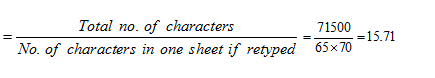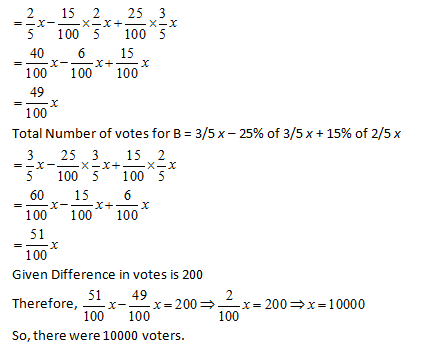Select Page

Introduction:
Percentage is one topic that appears underpins CAT Arithmetic essentially. It essentially is the first topic that you study in CAT Arithmetic and is the topic that finds near universal application in the whole area. In fact, right from our childhood, we have been calculating percentages in some form or the other and percentages form a ubiquitous part ofour everyday lives. By solving these five percentage questions, you learn the basic applications for this topic and how does this topic appear in different exams.

Question 1:A report consists of 20 sheets each of 55 lines and each such line consists of 65 characters. This report is reduced onto sheets each of 65 lines such that each line consists of 70 characters. The percentage reduction in number of sheets is closest to:
(a) 20%
(b) 5%
(c) 30%
(d) 35%

Option a

No. of Characters in one line = 65

No. of characters in one sheet = No. of lines × No. of characters per line = 55 × 65

Total number of characters = No. of sheets × No. of characters in one sheet = 20 × 55 × 65 = 71500

If the report is retyped –

New sheets have 65 lines, with 70 characters per line

No. of characters in one sheet = 65 × 70

Number of pages required,Hence, 16 pages will be required if report is retyped.

Hence, reduction of (20 – 16) = 4 pages

% reduction is = (4/20) x 100 = 20%

Question 2: The number of votes not cast for the PNC Party increased by 25% in the National General Election over those not cast for it in the previous Assembly Polls, and the PNC Party lost by a majority twice as large as that by which it had won the Assembly Polls. If a total 2,60,000 people voted each time, how many voted for the PNC Party in the previous Assembly Polls?
(a) 1,10,000
(b) 1,50,000
(c) 1,40,000
(d) 1,20,000

Option c

Let x voters voted against the party in the Assembly Poll.

Then votes in favour = 260000 – x

Therefore, majority of votes by which party won in previous poll = 260000– xx = 260000 – 2x

Next year votes against the PNC party increase by 25%

So, votes against the party in general election = 1.25 x

And votes polled in favour of the party = total votes  – votes against = 260000 – 1.25x

Therefore, majority of votes by which party lost in general election

= 1.25x – (260000 – 1.25x) = 2.5x – 260000

It is given that, PNC Party lost by a majority twice as large as that by which it had won the Assembly Polls, Therefore

2.5x – 260000 = 2(260000 – 2 x)

⇒ 2.5x – 260000 = 2 260000 – 4x

⇒ 6.5x = 3260000⇒x == 1,20,000

Therefore, votes polled by the voters for the party in Assembly Polls for previous year

= (2,60,000 – x) = (2,60,000 – 1,20,000) = 1,40,000.

Question 3: 2/5th of the voters promise to vote for A and the rest promised to vote for B. Of these, on the last day 15% of the voters went back of their promise to vote for A and 25% of voters went back of their promise to vote for B, and A lost by 200 votes. Then, the total number of voters is:
(a) 10000
(b) 11000
(c) 9000
(d) 9500

Option a

Let x be the total number of voters

Voters promised to A = 2/5 x

Voters backed out = 15% of 2/5 x

Voters promised to B = 3/5 x

Voters backed out = 25% of 3/5 x

Total Number of votes for A = 2/5 x – 15% of 2/5 x + 25% of 3/5 xQuestion 4: A person who has a certain amount with him goes to market. He can buy 50 oranges or 40 mangoes. He retains 10% of the amount for taxi fares and buys 20 mangoes and of the balance, he purchases oranges. Number of oranges he can purchase is:
(a) 36
(b) 40
(c) 15
(d) 20

Option d

The person can buy 50 oranges or 40 mangoes.

Let the price of one orange be Rs. x

Total amount the person has = Rs. 50x

40 mangoes cost 50x, So one mango costs 1.25x

10% of the total amount is retained for taxi fare = 10% of 50x = 5x

20 mangoes bought for 20 x 1.25x = 25x

Money left with the person = 50x – (Taxi fare) – (Mangoes cost)

= 50x – 5x – 25x = 20x

One Orange was for Rs. x, Therefore, 20 oranges can be bought with Rs. 20 x

Thus, the person bought 20 oranges.

Question 5: Forty per cent of the employees of a certain company are men and 75% of the men earn more than Rs. 25,000 per year. If 45% of the company’s employees earn more than Rs. 25,000 per year, what fraction of the women employed by the company earn Rs. 25,000 or less per year?
(a) 2/11
(b) 1/4
(c) 1/3
(d) 3/4

Option d

Let the total number of employees in the company be x

Then the number of men and women be 0.4x and 0.6x respectively.

75% of men earn more than Rs. 25000 => 0.75 x 0.4 x = 0.3 x

Total number of employees earning more than Rs. 25000 = 45% x = 0.45 x

Number of women earning more than Rs. 25000 =

Total employees earning more than Rs. 25000 – total number of Men earning more than Rs. 25000

= 0.45 x – 0.30 x = 0.15 x

Number of the women earning Rs. 25000 or less = 0.60 x – 0.15 x = 0.45 x

Fraction of the women employed by the company who earn Rs. 25000 or less

(0.45x/0.60x) = 45/60 = ¾

Alternate Solution:

Let there are 40 men and 60 women in the company. Now out of 40 men, 75% i.e. 30 earn more than Rs 25000 and 45% of the total employees i.e. 45 employees earn more than Rs 25000.

Hence, there are

45 – 30 = 15 women who earn more than Rs25000. So, 60 – 15 = 45 women earn less than Rs 25000.

Hence, the required fraction =  45/60 = ¾

Extra tips for CAT Percentage Questions
• The above questions illustrate the kind of problems that appear in MBA entrance exams. You can see from the problems above these questions are not purely based on the concept of percentages and require you to use some logic to identify the key considerations in the questions.
• Most of the CAT percentage questions will combine more than one topic in the problems and will require you to use some degree of logic to make equations in the problems.
• As with all CAT Arithmetic topics, the basic concepts (and when we say basic, we mean the absolute basic) can be found in school books.
• For bulk practicing problems for this topic, you can refer to RS Agarwal. Another good resource to practice problems and learn basic concepts for this topic is the Quantitative Aptitude book by Abhijit Guha.

Get Posts Like This Sent to your Email
Updates for Free Live sessions and offers are sent on mail. Don't worry: we do not send too many emails..:)
Get Posts Like This Sent to your Email
Updates for Free Live sessions and offers are sent on mail. Don't worry: we do not send too many emails..:)